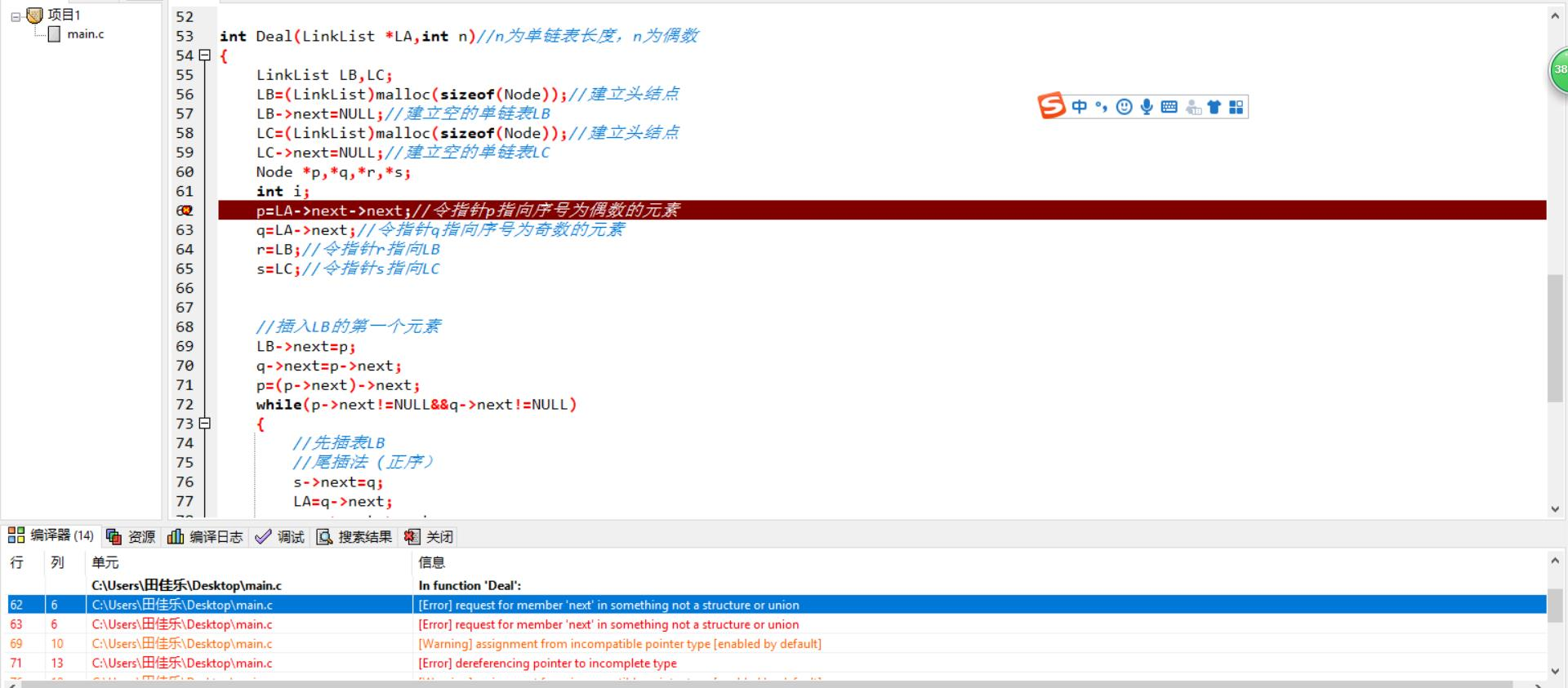【数据结构】指针和链表问题，求大佬解答`````` #include <stdio.h>
#include <stdlib.h>

#define MAXSIZE 20

typedef int ElemType;
typedef struct
{
ElemType data;
struct Node *next;

int main()
{
//建立单链表
LA->next=NULL;
Node *r,*s;
int c;
r=LA;
int flag=1,count=0;
while(flag)
{
printf("Please input new node to the LinkList,node must be intatger，input q to quit:\n");
scanf("%d",&c);
if(c!='q')
{
s=(Node*)malloc(sizeof(Node));
s->data=c;
r->next=s;
r=s;
count++;
}
else
{
flag=0;
r->next=NULL;
}
}
Deal(&LA,count);

//打印新生成的表
r=LA->next;
while(r->next!=NULL)
{
printf("%5d",r->data);
}
return 0;
}

{
LB->next=NULL;//建立空的单链表LB
LC->next=NULL;//建立空的单链表LC
Node *p,*q,*r,*s;
int i;
p=LA->next->next;//令指针p指向序号为偶数的元素
q=LA->next;//令指针q指向序号为奇数的元素
r=LB;//令指针r指向LB
s=LC;//令指针s指向LC

//插入LB的第一个元素
LB->next=p;
q->next=p->next;
p=(p->next)->next;
while(p->next!=NULL&&q->next!=NULL)
{
//先插表LB
//尾插法 (正序）
s->next=q;
LA=q->next;
q=q->next->next;
s=s->next;

//头插法（逆序）
p->next=LB->next;
LB->next=p;
p=p->next->next;
}

//合并LB/LC两表
if(p->next==NULL)
{
p->next=LC->next;
free(LC);
}
return 0;

}

``````

1个回答